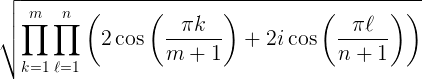# How Many Ways Can You Tile a Chessboard With Dominoes?

### When calculating the number of possible combinations, there's some math and, in turn, some code that can help you handle big figures.

· Big Data Zone · Code Snippet
Save
3.18K Views

suppose you have an n by m chessboard. how many ways can you cover the chessboard with dominoes?

it turns out there’s a remarkable closed-form solution:here are some questions you may have.

but what if n and m are both odd? you can’t tile such a board with dominoes.

yes, in that case, the formula evaluates to zero.

do you need an absolute value somewhere? or a floor or ceiling?

no. it looks like the double product could be a general complex number, but it’s real. in fact, it’s always the square of an integer.

does it work numerically?

apparently so. if you evaluated the product in a package that could symbolically manipulate the cosines, the result would be exact. in floating point, it cannot be, but at least in my experiments, the result is correct when rounded to the nearest integer. for example, there are 12,988,816 ways to tile a standard 8 by 8 chessboard with dominoes, and the following python script returns 12988816.0. for sufficiently large arguments the result will not always round to the correct answer, but for moderate-sized arguments, it should.

from numpy import pi, cos, sqrt

def num_tilings(m, n):
prod = 1
for k in range(1, m+1):
for l in range(1, n+1):
prod *= 2*cos(pi*k/(m+1)) + 2j*cos(pi*l/(n+1))
return sqrt(abs(prod))

print(num_tilings(8,8))


the code looks wrong. shouldn’t the ranges go up to  m  and  n  ?

no, python ranges are half-open intervals.  range(a, b)  goes from  a  to  b-1  . that looks unnecessarily complicated, but it makes some things easier.

you said that there was no need for absolute values, but you code has one.

yes, because while, in theory, the imaginary part will be exactly zero, in floating point arithmetic the imaginary part might be small but not zero.

where did you find this formula?

Topics:
big data, python, algorithm

Published at DZone with permission of John Cook, DZone MVB.

Opinions expressed by DZone contributors are their own.